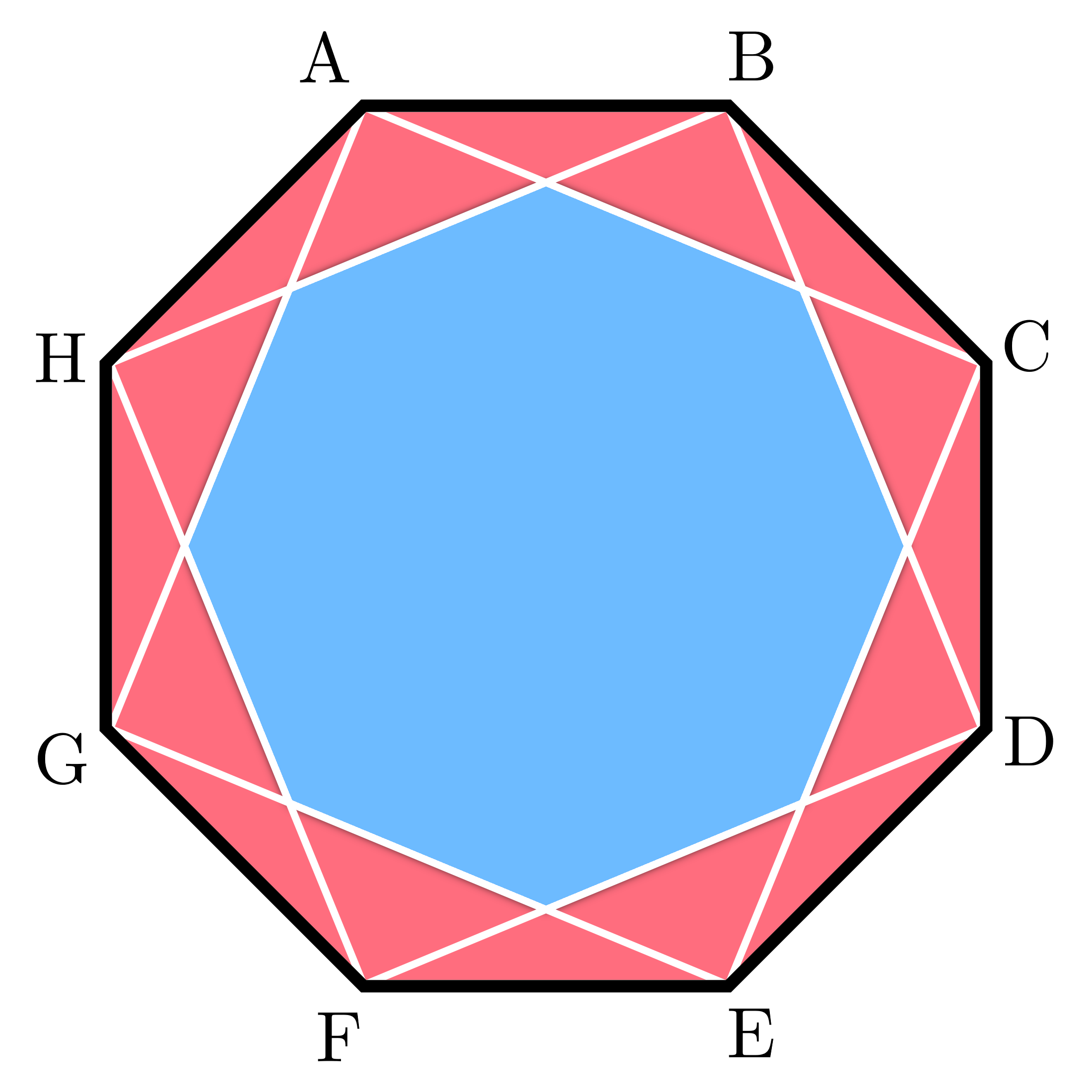# Octagon in an Octagon

Geometry Level 2A regular octagon $ABCDEFGH$ has squares $ACEG$ and $BDFH$ inscribed in it. These squares form a smaller octagon as shown.

Let the the area of octagon $ABCDEFGH$ be $A_L$ and the area of the smaller octagon be $A_S$. Then for some integers $a$ and $b$, where $b$ is square-free, $\large \dfrac{A_S}{A_L}=a-\sqrt{b}.$ Find $a+b$.

×